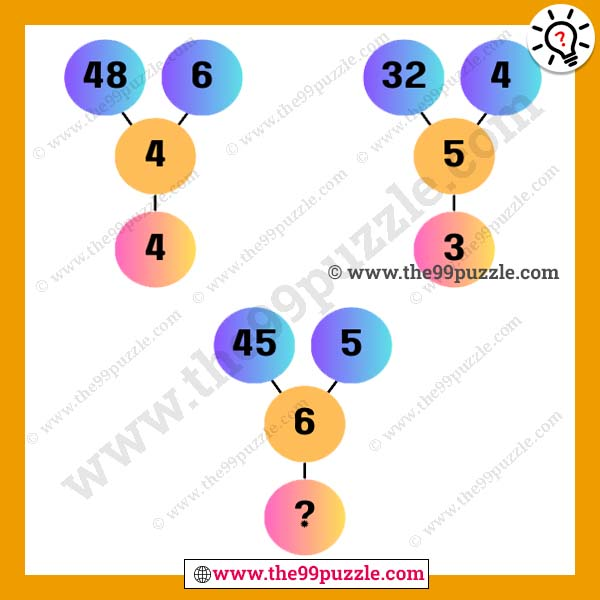# Genius puzzle of circle math with answer – Puzz280

Most challenging genius puzzle of circle math for school students. Here you will find various math puzzles that will sharpen your brain. In this tricky math puzzle, you will see three figures. All figures have the same math tricks. Now you have to crack the math logic and solve the last figure problem.  If you can practice these every day, you can solve any exam very quickly.###### Explanation:

1st Figure (Top Left) = 48÷6 = 8-4 = 4

2nd Figure (Top Right) = 32÷4 = 8-5 = 3

3rd Figure (Bottom) = 45÷5 = 9-6 = 3